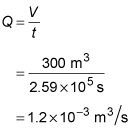##### Physics I: 501 Practice Problems For Dummies (+ Free Online Practice)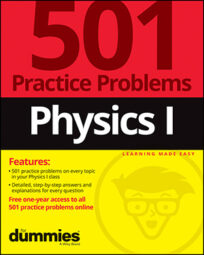Using physics, you can determine how the flow rate of a liquid is affected by cross-sectional area. For example, using the equation of continuity, given the flow rate and cross-sectional area of the opening to a water hose, you can calculate the speed at which the water exits the hose.

Here are some practice questions that you can try.

## Practice questions

1. Water travels through a hose at 0.8 meters per second. If the cross-sectional area of the exit nozzle is one-fifth that of the hose, at what speed does water exit the hose?

2. You are watching leaves float past you in a stream that is 0.76 meters wide. At one point, you estimate that the speed of the leaves triples. If the stream has a constant depth, what is the width of the stream at this point?

3. The volume flow rate through pipe 1 is 2.5 times that of pipe 2. If the cross-sectional area of pipe 1 is one-half that of pipe 2, what is the ratio of the flow speed in pipe 1 to that in pipe 2?

4. If the water that exits a pipe fills a pool that is 3 meters deep, 20 meters long, and 5 meters wide in 3 days, what is the flow rate?

The following are the answers to the practice questions:

1. 4.0 m/s

Use the equation of continuity: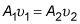You know that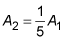and that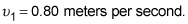Solve the continuity equation for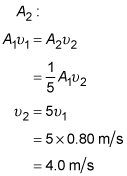2. 0.25 m

Use the equation of continuity:In terms of the stream width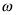and depth d, the cross-sectional area of the stream is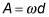Use this in the continuity equation: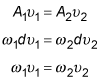You know that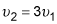so you can solve the continuity equation for the width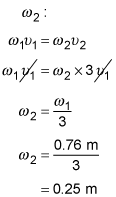3. 5.0

You know that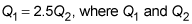are the volume flow rate through pipes 1 and 2, respectively. In terms of cross-sectional area and flow speed, the volume flow rate is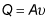When combined with the previous equation, you get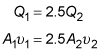You also know that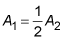Combine these equations to find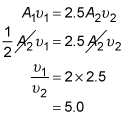4.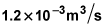Flow rate is the volume of fluid moving past a point per unit time. The volume of fluid that enters the pool is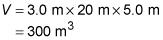The time it takes for this volume of water to enter the pool is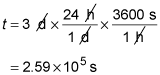The volume flow rate is therefore